American Journal of Theoretical and Applied Statistics
Volume 5, Issue 4, July 2016, Pages: 186-191

Cluster Analysis, K-Nearest Neighbour and Artificial Neural Network Applied to Credit Data to Classify Credit Applicants

Mutua Jennifer Ndanu1, Gichuhi Anthony Waititu2, Wanjoya Anthony Kiberia2, Muia Patricia Nthoki3

1Applied Statistics, Department of Statistics and Actuarial Science, Jomo Kenyatta University of Agriculture and Technology, Nairobi, Kenya

2Statistics, Department of Statistics and Actuarial Science, Jomo Kenyatta University of Agriculture and Technology, Nairobi, Kenya

3Education, Department of Educational, Administration and Planning, University of Nairobi, Nairobi, Kenya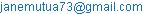(M. J. Ndanu)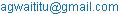(G. A. Waititu)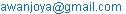(W. A. Kiberia)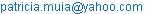(M. P. Nthoki)

Mutua Jennifer Ndanu, Gichuhi Anthony Waititu, Wanjoya Anthony Kiberia, Muia Patricia Nthoki. Cluster Analysis, K-Nearest Neighbour and Artificial Neural Network Applied to Credit Data to Classify Credit Applicants. American Journal of Theoretical and Applied Statistics. Vol. 5, No. 4, 2016, pp. 186-191. doi: 10.11648/j.ajtas.20160504.14

Received: May 5, 2016; Accepted: May 18, 2016; Published: June 7, 2016

Abstract: Potential risk on credit applicants is the probability of default on repayment of a credit facility rendered by a commercial bank. To improve efficiency in decision making on credit risk, therefore credit scoring models are developed. The objectives of this research areto classify credit applicants cluster analysis, Artificial Neural Network and K-Nearest neighbours techniques and to compare their predictive accuracy. The analysis was first by training the dataset, where by 70% of the data was used for training and the remaining 30% was used for testing. Finally, the ability of the developed models to forecast trends was investigated. Here we assume that a cluster is homogeneous, if it contains members that have a high degree of similarity. The analysis is therefore based on credit data provided by commercial banks in Kenya used to test the effectiveness of cluster analysis, K-Nearest neighbour (K-NN) and artificial neural network (ANN) models. To determine the best model in classification accuracy, confusion matrix was used. To test for the goodness of fit the chi square test was used. From the results of the study, the researcher concluded that ANN was better in predicting the classification of credit applicants than K-NN and Cluster Analysis.

Keywords: Cluster Analysis, ANN: Artificial Neural Network, K-NN: K-Nearest Neighbour, Credit Risk,
Overall Accuracy Rate, SSE: Sum of Square Errors

1. Introduction

1.1. Background of the Study

Commercial banks are significantly important to the economic development of a country, through the provision of credit services to their borrowers. The main idea behind banking is making profits from interest gained through provision of loans to its borrowers. Credit provision is the main income generating activity of commercial banks. However, it exposes the banks to credit risk. An accurate estimation of credit risk could be transformed into a more efficient use of economic capital.

Credit risk refers to the possibility of loss for a bank due to the inability of loan debtors to fulfillon time or completely their obligations they have assumed as part of their contracts with the bank (these obligations usually involve the repayment of principal debt and interest to the bank on predetermined dates). Therefore it is significant, screening the customer’s financial history and financial background before making any credit decision. Credit scoring is a technique that helps lenders to decide whether to grant credit to applicants with respect to the applicant’s characteristics. The objective of the credit scoring models is to determine credit applicant’s capacity to repay financial obligations by evaluating the credit risk of loan application, hence used to determine the credit granting decision between "bad" and"good" applicants which is a classification problem.

1.2. Statement of the Problem

Credit decisions have been a major problem in most of our commercial banks in Kenya. An incorrect decision made has a negative influence to the bank which could lead to economiclosses. Credit officers have been using some of the developed statistical models like lineardiscriminant analysis, logit and probit analysis, logistic regression but still they are faced withsome difficulties, hence they end up making inappropriate decisions. To improve on decision making was the need todevelop these three non-parametric classification models and compare them for their effectiveness in classification accuracy. With these models, we are able tonote their degree of correctness and to minimize type one and type two errors of classification. For cluster analysis, it can mine through the data and not only classify the data but alsoidentify other similar characteristics between the credit applicants. The developed models canbe consistently better in performance; hence will be applied best in the credit industry for betterdecision making and forecast of future trends. This minimizes the extent to which unexpectedlosses are incurred, improve the economic growth of the country and increase the profitabilityof our commercial bank.

1.3. Justification

In the past years, a number of effective credit scoring models have been developed. The credit departments have employed the use of these models but at some point they have failed in their decisions. This happens due to the highly nonlinear characteristic of credit data and the impact of economic conditions. Even the scoring models are accurate, some misclassification patterns could arise such as type one and type two errors due to the inconsistency of the data. Therefore this project aims at developing models by classification of data by cluster analysis, K-NN and ANN techniques. The choice of these techniques is due to their high applicability in pattern recognition. A comparison is made between these three models and the best is implemented. The credit departments would hence use the best developed model to make appropriate decisions on whether to grant or deny credit to its applicants.

1.4. Objectives

1.4.1. General Objective

The main aim of this project is to classify credit applicants using cluster analysis, Artificial Neural Network and K-Nearest Neighbors and to compare the predictive accuracy of these classification techniques.

1.4.2. Specific Objectives

To classify credit applicants using cluster analysis.

To classify credit applicants using Artificial Neural Network technique.

To classify credit applicants using K-Nearest Neighbour technique.

Tocompare the performance of these models to forecast trends.

1.5. Literature Review

Fisher  and Durand , were the first researchers on credit scoring who applied linear and quadratic discriminant analysis respectively to categorize credit applicants as "good" or "bad". Durand , was the first to recognize that credit officers should differentiate between good or bad loans by measurements of the applicants’ characteristics. After that, credit analysts in financial companies and mail order firms decide to whether to give loans or send merchandise.

Correa et al. , noted that using clusteranalysis as a predictive algorithm and then developing a scorecard for each of the resulting clusters is statistically better than building a single credit risk model for the entire population. On the other hand, it was also established thaton the task of cluster assignment for new applicants, the distance methodologies produce far superior results than the logistic regression and the MLP neural network models.

Fix and Hodges , were the first to propose K-NN as a nonparametric technique for classification. Findings showed that it was a suitable method of applying to consumer credit data due to its nonparametric nature that enabled modeling of irregularities in the risk function over the feature space. It performed better than other nonparametric techniques such as kernel methods when data is multidimensional.

Hand and Henley , found that K-NN technique performed better than other non-parametric methods such as kernel methods. Also in representing the accepted credit scoring techniques, K-NN achieved the lowest expected bad risk rate. They also noted that, the adjusted Euclidean metric led to an improvement over the standardEuclidean metric.

Khashman , employed neural networks to credit risk evaluation using the German dataset. Three neural network models with nine learning schemes were developed and then the different implementation outcomes were compared. The experimental results showed that one of the learning schemes achieved high performance with an overall accuracy rate of 83.6%.

Boguslauskas and Mileris  found that, rates of classification accuracy are very important in the estimation of quality of credit risk models. They created ANN models which showed that the rates of classification accuracy become higher when increasing time period of the data analyzed from 1 to 3 years. The highest accuracy was reached by the model that analyzed data of 3 years. The analysis of 4 years and more data reduced the value of the correct classification rate due to the network‘s over learning. Analyzing 3 year data of companies, 95.51% companies were classified correctly, the highest sensitivity reached by the model was 90.24% and specificity was 100%. This research confirmed that artificial neural networks are anefficient method for the estimation of credit risk in banks.

2. Methodology

In this section, we discuss basic algorithms used for cluster analysis, artificial neural network and k-nearest neighbor. Finally, we discuss model performance measures.

2.1. Training and Testing of the Data

For classification analysis using Cluster Analysis, ANN and K-NN, the dataset of 1500 credit applicants was first trained such that 70% of the data were used for training set, while the rest 30% were used for testing set. The training set was then used to develop the classification model, and the testing set later enhanced the validation process. The objective of training is to find the set of weights between the neurons that determine the global minimum of error function.

2.2. Cluster Analysis

Cluster analysis is a collection of statistical algorithms for investigating groups of samples that behave similarly or show similar characteristics. In addition, it is an unsupervised learning technique that identifies the complex relationships between variables, without imposing any restriction. All variables have the same importance, because the analysis goal is not to predict a certain value, but instead, to identify the presence of specific patterns or correlations among variables, to include the different variables into more homogenous groups.

Description of K-Means algorithm

K-mean some of the simplest unsupervised learning algorithms that solves the clustering problem whose objective is to classify.

Input the dataset of n observations X={x1,…, xn}, and choose the number of clusters k. randomly select the initial members for k cluster centers matrix V(0) for the dataset.

Using a distance function, assign each observation to the nearest cluster. For each observation xi, in each cluster cj, compute its classification function m (cj\xi). The classification function m(cj\xi) is the proportion of observation xi that belongs to cluster cj. The classification function is strict that is m(cj\xi)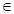{0, 1}

Cluster Validation

The evaluation of the resulting classification model is an integral part of the process of developing a classification model and there are well accepted evaluation measures and procedures like accuracy andcross-validation respectively. Each clustering algorithm defines its own type of cluster, thus require a different type of evaluation measure. In the case of kmeans clustering algorithm, clusters will be evaluated using SSE.

2.3. Artificial Neural Network

A neural network can be represented as a parallel connection of a set of nodes referred to as neurons, as defined by Gichuhi (2008). They are powerful tools for unknown data relationship modeling. ANNs able to recognize the complex pattern between input and output variables then predict the outcome of new Independent input data. The feed-forward neural network with back propagation (BP) is widely used for credit scoring, where the neurons receive signals from pre-layer and output them to the next layers without feedback. The nodes in input layer receive attributes values of each training sample and transmit the weighted outputs to hidden layer. The weighted outputs of the hidden layer are input to unit making up the output layer, which emits the prediction for given samples.

Definition of ANN

ANN constitutes of an input layer, hidden layer and an output layer. In this study we shall consider a feed forward neural network with m input nodes, one layer of t hidden nodes, one output node and an activation function (x). Weights Wpr, constitute the connection between the input and the hidden layer nodes for p{1,..., t} and r{0,.., m}. Wpo is the bias of the ith hidden node.

Weights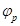, form the connection between the hidden layer and output layer for p{0,..., t}.

Input to the pth hidden node is the value

Ap(x) = Wpo+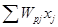(1)

Output to the pth hidden node is the value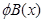(2)

Now input to the output node is the value

C(x) =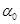+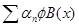(3)

Finally the output is the value

D(x) = D(x)                                     (4)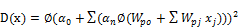(5)

The output D(x) is the final classifier of the credit applicants, defined by its estimator,

D(x)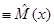(6)

Being a classification problem, the estimator will be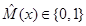.

2.4. K-Nearest Neighbor

K-NN is a non-parametric classification technique which stores all available cases and classifiesnew cases based on a similarity measure. A training data set is collected, for this training dataset, a distance function is introduced between the explanatory variable of observations. For eachnew observation this method explores the pattern space for the K-NN that isclosest to thenew observation in term of distance between the explanatory variables. The new observationis assigned by a majority vote of its neighbors, with the observation being assigned to the classmost common amongst its k nearest neighbors measured by a distance function.

2.4.1. Definition of K-NN

Input D, the set of training objects, z, the test object, which is a vector of attribute values

And, L, the set of classes used to label the objects. For each object yD, compute d (z, y), the distance between z and y. Select N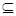D, the set of neighborhood of k closest training objects for z. Output, CzL, the class of z. The output is the classification function which is strictly{0, 1}.

2.4.2. Parameter Selection

The best choice of k depends upon the data; generally, larger values of k reduce the effect of noise on the classification, but make boundaries between classes less distinct. The choice of the number of nearest neighbors chosen (k) determines the bias/variance trade-off in the estimator. The k has to be much smaller than the smallest class. A study by Enas and Choi  suggested that k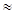n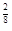orn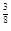is reasonable.

2.5. Criteria of Model Performance Evaluation

In credit risk classification, even a small improvement in predictive accuracy can translate into significant savings. The very significant importance of credit risk in banking sector determines demand to reach as high as possible credit risk estimation accuracy. Thus, we will evaluate the rates of classification accuracy using confusion matrix and the goodness of fit using chi-square test.

Confusion Matrix

The confusion matrix also known as the classification matrix is a standard tool for evaluationof statistical models forprediction accuracy. It allows visualization of the performance of a model. The rows in the matrix represent the predicted values for the model, whereas the columns represent the actual values. Classification matrix sorts all cases from the model into categories, by determining whether the predicted value corresponds to the actual value.

Table 1. Confusion matrix.

 Outcomes TRUE FALSE TRUE a [TP] b [FP] Predicted FALSE c [FN] d [TN]

Classification accuracy rate is the common measure used in evaluating the predictive accuracy of classification models, since it represents the percentage of applications that are classified correctly, Abdou et al. . Other measures are Specificity and sensitivity.

Correct Classification=Specificity=Sensitivity=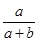2.6. Data Analysis

Oso and Onen  define data analysis as the organization, interpretation and presentation of collected data. Data will be analyzed using the R-GUI statistical software. Data will be loaded into the software. The researcher will then train the data using function caret and fit a cluster analysis, ANN and K-NN models. To assess the goodness of fit of these models chi square test will be used. Forprediction accuracy classification matrix will be used.

3. Results

3.1. Predictive Ability of the Cluster Analysis Model

The validation process will be used to help select the proper number of clusters, using a silhouette plot. An average silhouette width value of 0.93 was obtained for a two cluster solution. This showed that there is a good structure to thetwo clusters, with most observations seeming to belong to the cluster that they have been classified in. To develop the confusion matrix, the observed defaults of zero’s and one’s were also classified into clustersto enable easy comparison to its model clusters solution.

Table 2. Confusion matrix for the Cluster Analysis classification model.

 References 0 1 0 273 26 Predicted 1 147 4

Table 2 summarizes the classification capability of the cluster Analysis model. From these statistics, a specificity value of 26.49% was obtained which represented the percentage of the rejected applications that were classified correctly, a sensitivity value of 91.30%was obtained which represented the percentage of the accepted applications were classified correctly and the overall correct classification rate of the cluster Analysis model was 61.56% with a 0.5 threshold. Other values obtained were omission rate value of 0.3377246, AUC value was 0.521629 and akappa statistic value of -0.075245.

3.2. Predictive Ability of the ANN Classification Model

Using the dataset, the value of the mean square error (MSE) was used to determine the optimal number of hidden nodes in our neural network. The number of hidden nodes is based on the minimum mean square error obtained. This study thus settled on seven hidden nodes as shown in figure 1.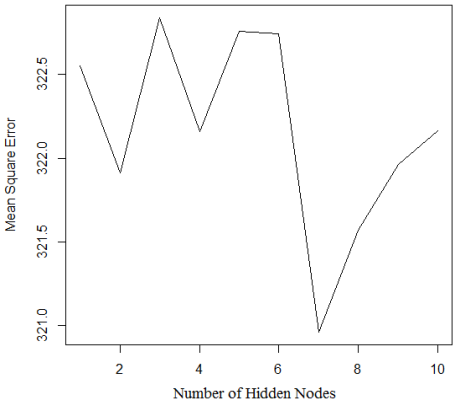Figure 1. Determining the Number of Hidden Nodes.

To test the ability of the model in classification, a confusion matrix was developed.

Table 3. Confusion matrix of the ANN classification model.

 References 0 1 0 218 23 Predicted 1 65 14

From Table 3, the measures of predictive power were calculated. Sensitivity, which was the proportion of credit applicants with value zero which were correctly classified, was 0.8622754. Specificity, which was the proportion of credit applicants with value one which were correctly classified was 0.770318. Accuracy, which was the proportion of predictions that were correctly classified, was 0.8044444. Other values obtained given a threshold of 0.5 were: Omission ratevalue was 0.1377246, AUC value was 0.8162967 and kappa statistic value was 0.6015856.

3.3. Predictive Ability of the K-NN Classification Model

The selection of the appropriate tuning parameter size, k, is by trial and error. But the researcher decided to fit a K-NN model to size fifteen, suggested by Enas and Choi  as k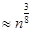. The results obtained from the classifying model for credit applicants are given in the table 4.

Table 4. Confusion matrix of the K-NN classification model.

 References 0 1 0 259 87 Predicted 1 39 65

From these statistics in table 4, the measures of predictive power were calculated. Specificity, which is the proportion of credit applicants with value one which were correctly classified was 0.4276316. Sensitivity, which was the proportion of credit applicants with value zero which were correctly classified, was 0.8691275. Accuracy, which was the proportion of predictions that were correctly classified, was 0.72. Other values obtained given a threshold of 0.5 were: Omission rate valuewas 0.5723684, AUC value was 0.6483795 and kappa statistic value was 0.3216405.

3.4. Comparison of the Classification Models

The predictive power of the models was accessed using confusion matrix and chi-square test. Table 5 lists out various model evaluation parameters of the three classification models.

Table 5. Model Evaluation Parameters.

 Specifity Sensitivity Accuracy Kappa Cluster 0.265 0.913 0.62 -0.075 ANN 0.77 0.862 0.804 0.602 K-NN 0.869 0.428 0.72 0.322

From the Kappa statistics, it is inferred that the ANN models shows a Substantial degree of agreement having a kappa statistic value of 0.6015856 compared to K-NN model with kappa statistic value of 0.3216405 showing a fair agreement and cluster analysis with kappa statistic value of -0.075 showing no agreement. For predictive accuracy in classifying credit applicants, the ANN model had a higher classification accuracy of 80.4% compared to K-NN model with an accuracy of 72% and then cluster analysis with an accuracy of 62%. In fact, Cluster Analysis model performed better when classifying accepted applications (91.30%) than classifying bad applications (26.50%). Similarly, ANN model performed moderately when classifying accepted applications (86.2%) than classifying bad applications (77%). But, K-NN model performed better when classifying bad applications (86.9%) than classifying accepted applications (42.8%).

Chi square test statistic was also used to test the goodness of fit whose values are shown in table 6.

Table 6. Results from the chi square tests.

 Chi Square Test Values Model P-Value Cluster Analysis 0.9033 ANN 0.4778 K-NN 0.5189

The p-values obtained are greater than 0.05. Hence there was no sufficient evidence to reject the null hypothesis. This implies that for all the models, the observed values and the expected values were almost the same hence a good classification was done.

4. Conclusion and Recommendations

4.1. Conclusion

One objective of this study was to compare their predictive accuracyin classification of credit applicants. ANNmodel, K-NN model and Cluster Analysis modelcan effectively be used in classification of credit applicants. ANN performed better than K-NN and Cluster Analysis considering the overall correct classification. On the other hand, the K-NN model outperformed the ANN and Cluster analysis model in screening rejected applications, identifying potential defaulters and henceminimizing Type II error. Goodness of fit was done and all models were found to have beensignificant. Hence, ANN is considered as the best model in this study.

4.2. Recommendations

From the study, some issues related to the choice of a similarity or dissimilarity and standardization measures should be considered. Also in predicting the accuracy of classification ofcredit applicants using cluster analysis the data should be grouped into clusters to enable easemanipulation. The reliability and external validity of a cluster solution must be considered.

References

1. Abdou, H, J Pointon and A El-Masry (2007), ‘On the applicability of credit scoringmodels in Egyptian banks’, Banks Bank Syst 2(1), 4–19.
2. Bekhet, H and S Eletter (2012), ‘Credit risk management for the Jordanian commercial banks: a business intelligence approach’, Aust. J. Basic Appl. Sci6(18), 188–195.
3. Boguslauskas, V and R Mileris (2009), ‘Corporate distress diagnosis: Comparisons using linear discriminant analysis and neural networks (the italian experience)’, Economics of engineering decisions.
4. Correa, A, A Gonzalez, C Nieto and D Amezquita (2012), Constructing a Credit Risk Scorecard using Predictive Clusters, SAS Global Forum.
5. Durand, D (1941), Risk elements in consumer instalments financing, New York: national bureau of economic research.
6. Enas, G G and S C Choi (1986), ‘Choice of the smoothing parameter and efficiency of k-nearest Neighbor classification’, Computers and Mathematics with Applications 12A (2), 235–244.
7. Fisher, R A (1936), ‘The use of multiple measurement in taxonomic problems’, Annals ofEugenic 7, 179–188.
8. Fix, E and J Hodges (1952), Discrimatory analysis; nonparametric discrimination: consistency properties, report 4, project 21-49-004 edn, us airforce school of aviation medicine, random Field.
9. Glorfeld, LWand B C Hardgrave (1996), ‘an improved method for developing neural networks: the case of evaluating commercial loan credit worthiness’, Computers and Operations Research 23 (10), 933–944.
10. Hand, D J and W E Henley (1996), ‘A k-nearest neighbour classifier for assessing consumer credit risk’, the statistician 45 (1), 77–95.
11. Khashman, A (2010), ‘Neural network for credit risk evaluation: investigation of different neural Models and learning schemes.)’, Exp. Syst. Appl. 37 (9), 6233–6239.
12. Oso, W Y and D Onen (2009), ‘A guide line to writing a research proposal and report’, A Handbook of Beginning Researchers.

 Contents 1. 1.1. 1.2. 1.3. 1.4. 1.5. 2. 2.1. 2.2. 2.3. 2.4. 2.5. 2.6. 3. 3.1. 3.2. 3.3. 3.4. 4. 4.1. 4.2.
Article ToolsAbstractPDF(340K)